# keras-unet

Showing:

0

242

7mos ago

6

## Package

0

MIT

### Categories

Helper package with multiple U-Net implementations in Keras as well as useful utility tools helpful when working with image segmentation tasks

# Features:

• U-Net models implemented in Keras
• Utility functions:
• Plotting images and masks with overlay
• Plotting images masks and predictions with overlay (prediction on top of original image)
• Plotting training history for metrics and losses
• Cropping smaller patches out of bigger image (e.g. satellite imagery) using sliding window technique (also with overlap if needed)
• Plotting smaller patches to visualize the cropped big image
• Reconstructing smaller patches back to a big image
• Data augmentation helper function
• Notebooks (examples):
• Training custom U-Net for whale tails segmentation
• Semantic segmentation for satellite images
• Semantic segmentation for medical images ISBI challenge 2015

# Installation:

``````pip install git+https://github.com/karolzak/keras-unet
``````

or

``````pip install keras-unet
``````

# Usage examples:

• U-Net implementations in Keras:
• Utils:
``````- [Plot training history](#Plot-training-history)
- [Get smaller patches/crops from bigger image](#Get-smaller-patches/crops-from-bigger-image)
- [Plot small patches into single big image](#Plot-small-patches-into-single-big-image)
- [Reconstruct a bigger image from smaller patches/crops](#Reconstruct-a-bigger-image-from-smaller-patches/crops)
``````

### Vanilla U-Net

Model scheme can be viewed here

``````from keras_unet.models import vanilla_unet

model = vanilla_unet(input_shape=(512, 512, 3))
``````

[back to usage examples]

### Customizable U-Net

Model scheme can be viewed here

``````from keras_unet.models import custom_unet

model = custom_unet(
input_shape=(512, 512, 3),
use_batch_norm=False,
num_classes=1,
filters=64,
dropout=0.2,
output_activation='sigmoid')
``````

[back to usage examples]

### U-Net for satellite images

Model scheme can be viewed here

``````from keras_unet.models import satellite_unet

model = satellite_unet(input_shape=(512, 512, 3))
``````

[back to usage examples]

### Plot training history

``````history = model.fit_generator(...)

from keras_unet.utils import plot_segm_history

plot_segm_history(
history, # required - keras training history object
metrics=['iou', 'val_iou'], # optional - metrics names to plot
losses=['loss', 'val_loss']) # optional - loss names to plot
``````

Output: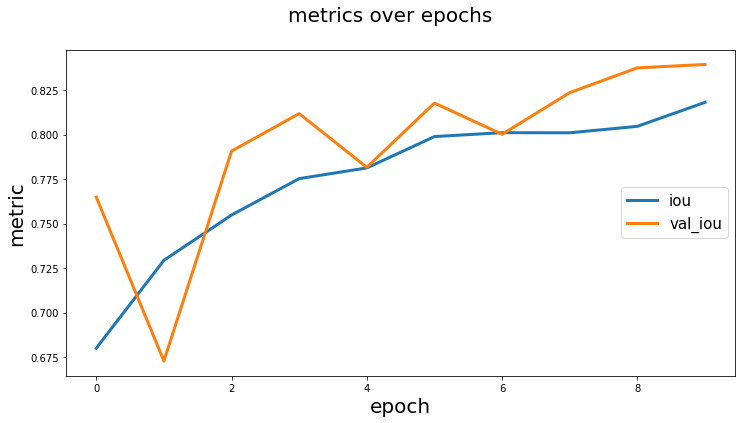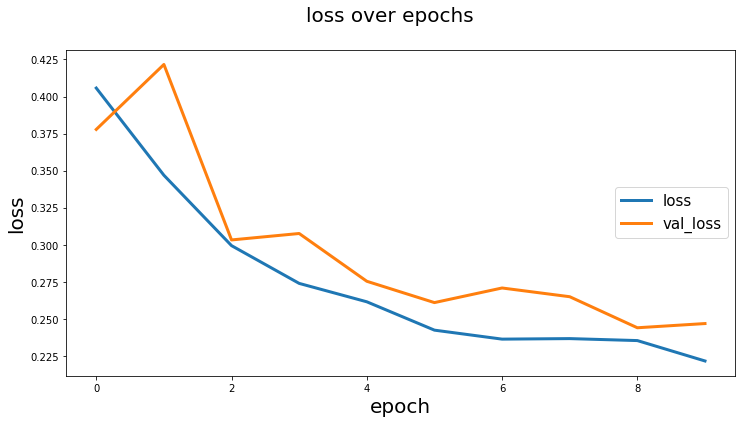[back to usage examples]

### Plot images and segmentation masks

``````from keras_unet.utils import plot_imgs

plot_imgs(
org_imgs=x_val, # required - original images
pred_imgs=y_pred, # optional - predicted masks
nm_img_to_plot=9) # optional - number of images to plot
``````

Output: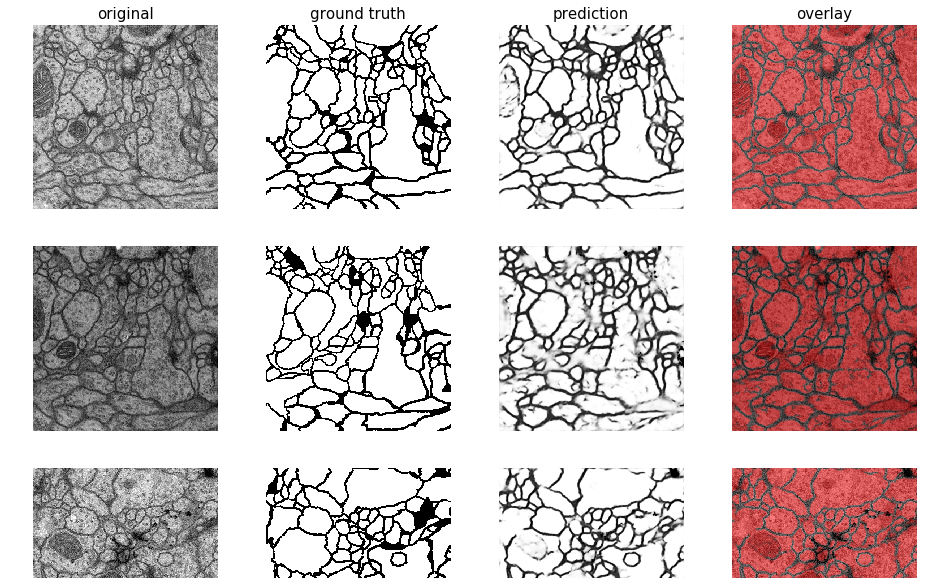[back to usage examples]

### Get smaller patches/crops from bigger image

``````from PIL import Image
import numpy as np
from keras_unet.utils import get_patches

x = np.array(Image.open("../docs/sat_image_1.jpg"))
print("x shape: ", str(x.shape))

x_crops = get_patches(
img_arr=x, # required - array of images to be cropped
size=100, # default is 256
stride=100) # default is 256

print("x_crops shape: ", str(x_crops.shape))
``````

Output:

``````x shape:  (1000, 1000, 3)
x_crops shape:  (100, 100, 100, 3)
``````

[back to usage examples]

### Plot small patches into single big image

``````from keras_unet.utils import plot_patches

print("x_crops shape: ", str(x_crops.shape))
plot_patches(
img_arr=x_crops, # required - array of cropped out images
org_img_size=(1000, 1000), # required - original size of the image
stride=100) # use only if stride is different from patch size
``````

Output:

``````x_crops shape:  (100, 100, 100, 3)
``````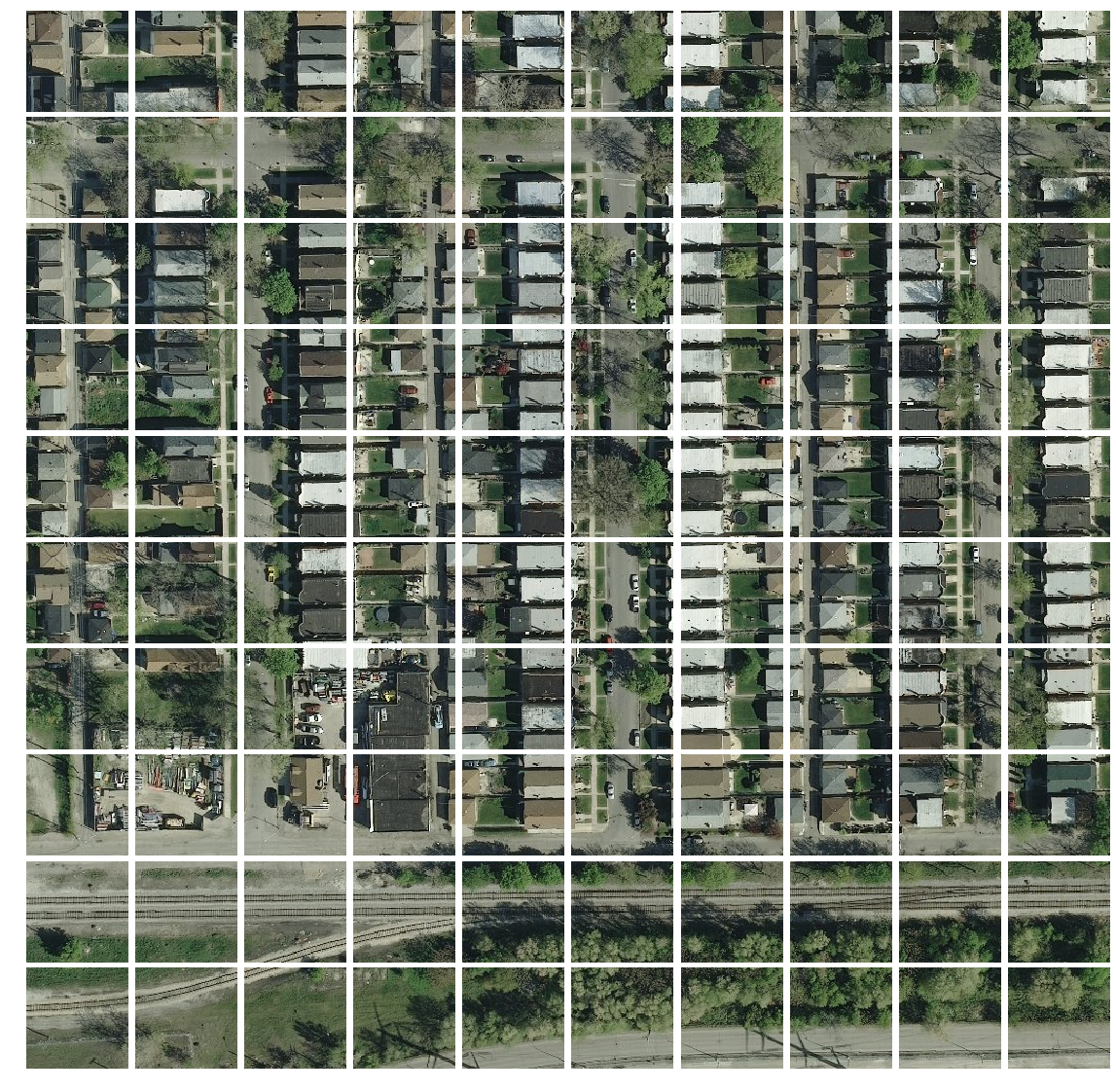[back to usage examples]

### Reconstruct a bigger image from smaller patches/crops

``````import matplotlib.pyplot as plt
from keras_unet.utils import reconstruct_from_patches

print("x_crops shape: ", str(x_crops.shape))

x_reconstructed = reconstruct_from_patches(
img_arr=x_crops, # required - array of cropped out images
org_img_size=(1000, 1000), # required - original size of the image
stride=100) # use only if stride is different from patch size

print("x_reconstructed shape: ", str(x_reconstructed.shape))

plt.figure(figsize=(10,10))
plt.imshow(x_reconstructed)
plt.show()
``````

Output:

``````x_crops shape:  (100, 100, 100, 3)
x_reconstructed shape:  (1, 1000, 1000, 3)
``````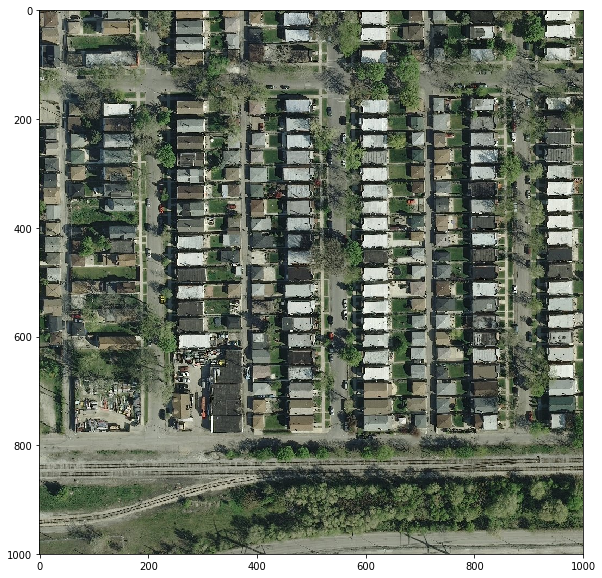[back to usage examples]

## Rate & Review

Great Documentation0
Easy to Use0
Performant0
Highly Customizable0
Bleeding Edge0
Responsive Maintainers0
Poor Documentation0
Hard to Use0
Slow0
Buggy0
Abandoned0
Unwelcoming Community0
100